## The Mrs. White probability puzzle

tl;dr -I don’t remember how many games of Clue I’ve played but I do remember being surprised by Mrs White being the murderer in only 2 of those games. Can you give an estimate and an upper bound for the number of games I have played?
We solve this problem by using Bayes theorem and discussing the data generation mechanism, and illustrate the solution with R.The characters in the original game of Clue. Mrs White is third from the left on the first row (and is now retired!)

#### Making use of external information with Bayes theorem

Having been raised a frequentist, I first tried to solve this using a max likelihood method, but quickly gave up when I realized how intractable it actually was, especially for the upper bound.
This is a problem where conditioning on external knowledge is key, so the most natural way to tackle it is to use Bayes theorem. This will directly yield an interpretable probability for what we’re looking for (most probable number of and uncertainty interval)

Denote an integer n>3 and: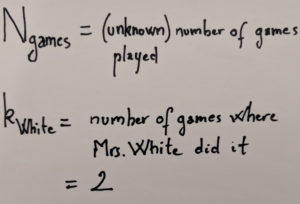Our notations for the problem

What we want writes as a simple product of quantities that we can compute, thanks to Bayes: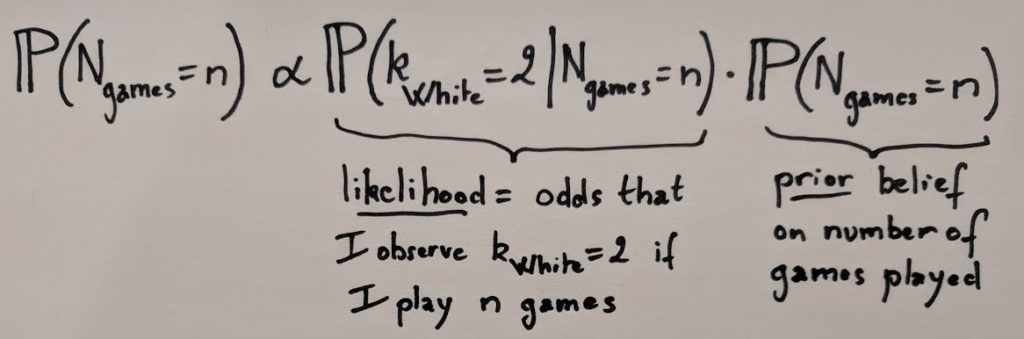Bayes theorem gives us directly the probability we are looking for
Notice that there is an “proportional to” sign instead of an equal. This is because the denominator is just a normalization constant, which we can take care of easily after computing the likelihood and the prior.
###### Likelihood
The likelihood indicates the odds of us observing the data (in this case, that k_Mrs_White = 2) given the value of the unknown parameter (here the number of games played). Since at the beginning of each game the murderer is chosen at uniform random between 6 characters, the number of times Mrs White ends up being the culprit can be modeled as a binomial distribution with parameters n and 1/6.

This will be easily obtained using the dbinom function, which gives directly the exact value of P(x = k), for any x and a binomial distribution of parameters n and p. Let’s first import a few useful functions that I put in our GitHub repo to save some space on this post, and set a few useful parameters:
```library(tidyverse)
source("clue/clue_functions.R")

## Parameters
k_mrs_white <- 2 # Number of times Mrs. White was the murderer
prob <- 1/6 # Probability of Mrs. White being the murderer for one game
```
Note that we can’t exactly obtain the distribution for any game from 1 to infinity, so we actually compute the distribution for 1 to 200 games (this doesn’t matter much in practice):
```x <- 1:200 # Reduction of the problem to a finite number of games

## Likelihood
dlikelihood <- dbinom(k_mrs_white, x, prob)
```
easy enough 🙂

Side note: when I was a student, I kept forgetting that the distribution functions existed in R and whenever I needed them I used to re-generate them using the random generation function (rbinom in this case) 🤦‍♂️
###### Prior
There are a lot of possible choices for the prior but here I’m going to consider that I don’t have any real reason to believe and assume a uniform probability for any number of games between 3 and 200:
```dprior1 <- dunifdisc(x,3,100)
plot_clue_prior(x, dprior1)
```Uniform prior for all number of games between 3 and 100
###### First posterior
Using the likelihood and the prior, we can easily compute the posterior, normalize it and plot it:
```dposterior1 <- dlikelihood * dprior1
dposterior1 <- dposterior1 / sum(dposterior1)
plot_clue_posterior(x, dposterior1)
```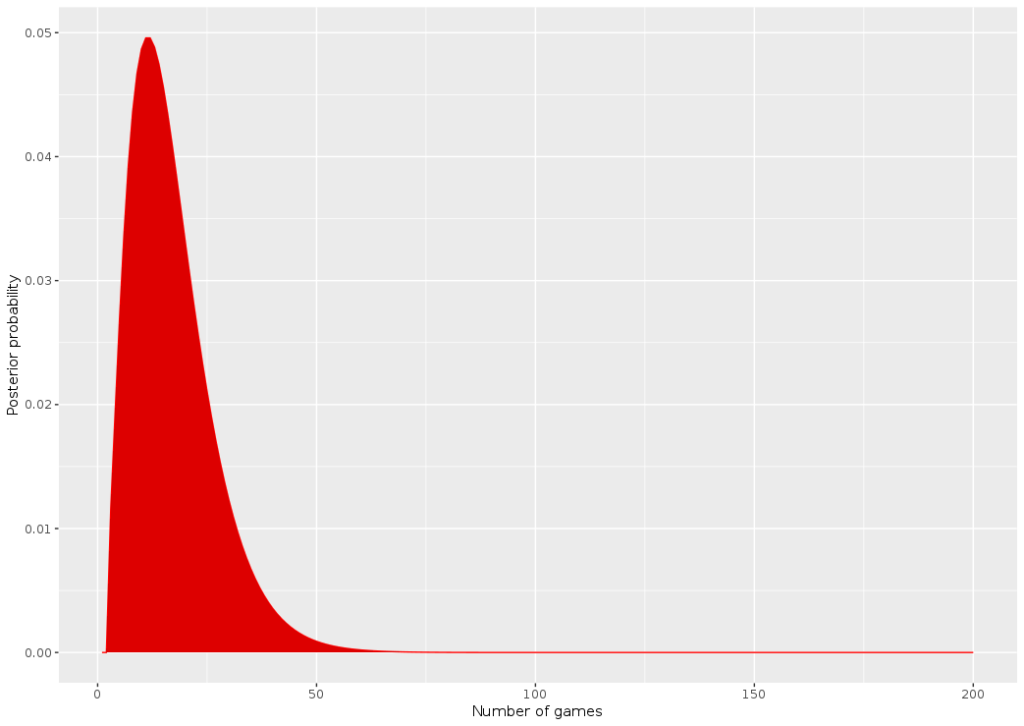Plot of the first posterior computed
We can also compute directly the estimates we’re looking for. The most probable number of games played is 11:
```> which.max(dposterior1)
 11
```
And there is a 95% chance that the number of games is less than 40:
```> threshold_val <- 0.975
> which(cumsum(dposterior1) > (threshold_val))
 40
```

#### A more realistic data generation mechanism

I find this result very unsatisfying. It doesn’t “feel” right to me that someone would be surprised by only 2 occurrences of Mrs White being guilty in such a small number of games! For example, I simulated 40 games, a number that was supposedly suspiciously high according to the model:
```set.seed(9)
N_sim_games <- 40
sim_murderer <- runifdisc(N_sim_games, 6)

plot_murderer <- ggplot(tibble(x=1:N_sim_games, y=sim_murderer), aes(y, stat(count))) +
geom_histogram(aes(y =..count..),
bins=6, fill="white",colour="black") +
ylab("Frequency - murderer") +
xlab("Character #") +
scale_x_continuous(breaks = 1:6)
print(plot_murderer)
```Simulating 40 games of Clue. Character #4 and #5 were the murderer in respectively only 2 and 3 games
We observe that characters #4 and #5 are the murderers in respectively only 2 and 3 games!

In the end I think what really counts is not the likelihood that *Mrs White* was the murderer 2 times, but that the *minimum* number of times one of the characters was the culprit was 2 times!

I think it’s a cool example of a problem where just looking at the data available is not enough to make good inference – you also have to think about *how* the data was generated (in a way, it’s sort of a twist of the Monty Hall paradox, which is one of the most famous examples of problems where the data generation mechanism is critical to understand the result).

I wrote a quick and dirty function based on simulations to generate this likelihood, given a certain number of games. I saved the distribution directly in the GitHub (and called it Gumbel since it kinda looks like an extreme value problem) so that we can call it and do the same thing as above:
```gumbelclue_2 <- readRDS("clue/dcluegumbel_2.rds")
gumbelclue_2 <- gumbelclue_2[x]

dposterior_gen <- gumbelclue_2 * dprior1
dposterior_gen <- dposterior_gen / sum(dposterior_gen)

plot_clue_posterior(x, dposterior_gen)
```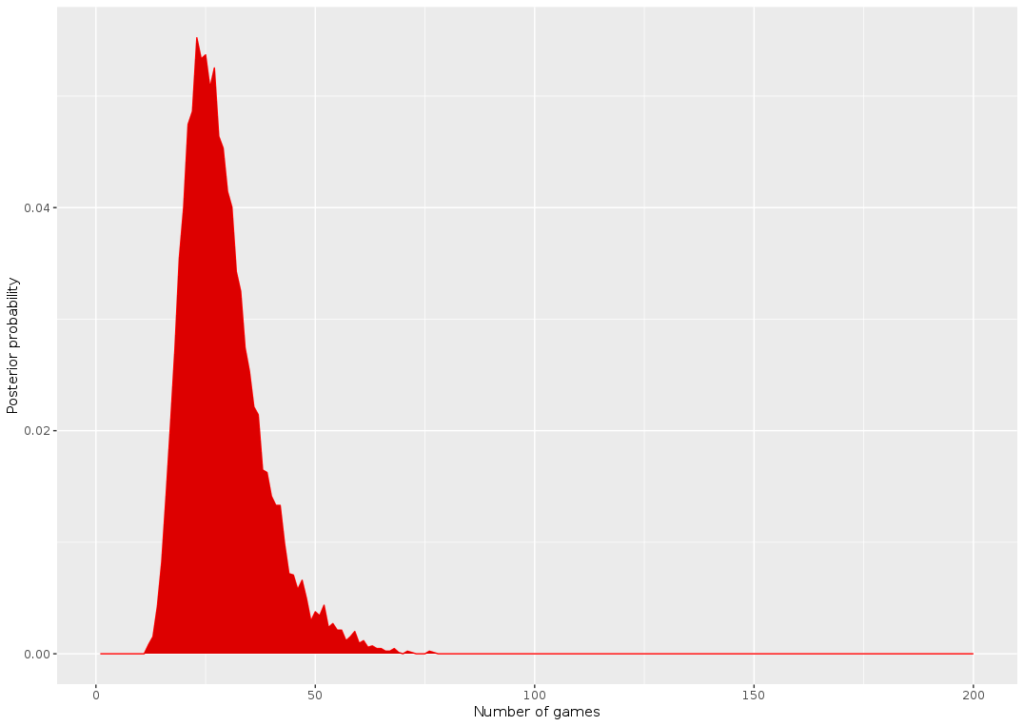Posterior with the new data generation mechanism
The new posterior has the same shape but appears shifted to the right. For example N_games = 50 seems much more likely now! The estimates are now 23 for the number of games:
```> which.max(dposterior_gen)
 23
```
And 51 for the max bound of the uncertainty interval
```> threshold_val <- 0.975
> which(cumsum(dposterior_gen) > (threshold_val))
 51```

Credits for title image: Yeonsang

## Ranking places with Google to create maps

Today we’re going to use the googleway R package, which allows their user to do requests to the GoogleMaps Places API. The goal is to create maps of specific places (restaurants, museums, etc.) with information from Google Maps rankings (number of stars given by other people). I already discussed this in french here to rank swimming pools in Paris. Let’s start by loading the three libraries I’m going to use : googleway, leaflet to create animated maps, and RColorBrewer for color ranges.

```library(googleway)
library(leaflet)
library(RColorBrewer)
```

First things first. To do API request to Google, we need an API key ; you can ask for one here. We’ll use this key for the rest of the program, so let’s declare a global variable :

```api_key <- "YourKeyHereIWontGiveYouMine"
```

## Retrieving Google Data

We’re going to use the google_places function to get a list of places matching a description, called research in my program (for instance : “Restaurant, Paris, France”). The output are multiple, and I’m going to store the place ID and the rating. I’ll also store the research token ; that’ll be explained later.

```gmaps_request <- google_places(search_string = research, language = language, key = api_key)
gmaps_data <- gmaps_request\$results

place_id <- gmaps_data\$place_id
rating <- gmaps_data\$rating

token <- gmaps_request\$next_page_token
```

This function returns up to 20 places associated to the research by Google. If you want more than 20, you need to use the token previously stored in order to ask the Google Places API to give you the next results, by tweaking the function this way :

```gmaps_request <- google_places(search_string = research, language = language, key = api_key, page_token = token)
```

There are tow caveats to this function. Firstly, the token can be NULL. In this case, there isn’t any further research results you can get. This happens automatically as soon as you reach 60 results. Secondly, the API needs time to refresh the token research (see here) ; that’s why we’re going to make R wait a few seconds, using Sys.sleep(time) between our requests. Our complete function is therefore :

```gmaps_request <- google_places(search_string = research, language = language, key = api_key)
gmaps_data <- gmaps_request\$results

place_id <- gmaps_data\$place_id
rating <- gmaps_data\$rating

token <- gmaps_request\$next_page_token
Sys.sleep(5)
continue <- TRUE

while (continue) {

gmaps_request <- google_places(search_string = research, language = language, key = api_key, page_token = token)
gmaps_data <- gmaps_request\$results

if (!is.null(gmaps_request\$next_page_token)) {
place_id = c(place_id,gmaps_data\$place_id)
rating = c(rating,gmaps_data\$rating)
token <- gmaps_request\$next_page_token
Sys.sleep(5)
}
else{continue <- FALSE}
}
```

Now we’re going to search for the spatial coordinates of the places we found. To this extent, we’re going to use the google_place_details function of the packages, and retrieve latitude and longitude with these two functions :

```get_lat <- function(id, key, language) {
id <- as.character(id)
details <- google_place_details(id, language = language, key=key)
return(details\$result\$geometry\$location\$lat)
}

get_lng <- function(id, key, language) {
id <- as.character(id)
details <- google_place_details(id, language = language, key=key)
return(details\$result\$geometry\$location\$lng)
}
```

All these blocks add up to build the complete function :

```get_gmaps_data <- function(research, api_key, language) {

gmaps_request <- google_places(search_string = research, language = language, key = api_key)
gmaps_data <- gmaps_request\$results

place_id <- gmaps_data\$place_id
rating <- gmaps_data\$rating

token <- gmaps_request\$next_page_token
Sys.sleep(5)
continue <- TRUE

while (continue) {

gmaps_request <- google_places(search_string = research, language = language, key = api_key, page_token = token)
gmaps_data <- gmaps_request\$results

if (!is.null(gmaps_request\$next_page_token)) {
place_id <- c(place_id, gmaps_data\$place_id)
rating <- c(rating, gmaps_data\$rating)
token <- gmaps_request\$next_page_token
Sys.sleep(5)
}
else{continue <- FALSE}
}

lat = sapply(place_id, get_lat, key=api_key, language=language)
lng = sapply(place_id, get_lng, key=api_key, language=language)

return(data.frame(place_id, rating, lat, lng))
}
```

## Map plot

The next part is more classical. We’re going to order the ratings of the data frame built by the previous function in order to arrange the places in differents groups. Each of the groups will be associated to a color on the data plot. If we want to make number_colors groups with the color scheme color (for instance, “Greens”), we are using the following instructions :

```color_pal <- brewer.pal(number_colors, color)
pal <- colorFactor(color_pal, domain = seq(1,number_colors))

plot_data <- gmaps_data
plot_data\$ranking <- ceiling(order(gmaps_data\$rating)*number_colors/nrow(plot_data))
```

The definitive function just needs the addition of the leaflet call :

```show_map <- function(number_colors, gmaps_data, color="Greens") {

color_pal <- brewer.pal(number_colors,color)
pal <- colorFactor(color_pal, domain = seq(1,number_colors))

plot_data <- gmaps_data
plot_data\$ranking <- ceiling(order(gmaps_data\$rating)*number_colors/nrow(plot_data)) leaflet(plot_data) %>% addTiles() %>%
addCircleMarkers(
radius = 6,
fillColor = ~pal(ranking),
stroke = FALSE, fillOpacity = 1
) %>% addProviderTiles(providers\$CartoDB.Positron)
}
```

## Examples

I just need to combine these two functions in one, and then to do some food-related examples !

```maps_ranking_from_gmaps <- function(research, api_key, language, number_colors=5, color="Greens") {
show_map(number_colors, get_gmaps_data(research, api_key, language), color)
}

maps_ranking_from_gmaps("Macaron, Paris, France", api_key, "fr")
maps_ranking_from_gmaps("Macaron, Montreal, Canada", api_key, "fr")

maps_ranking_from_gmaps("Poutine, Montreal, Canada", api_key, "fr", 5, "Blues")
maps_ranking_from_gmaps("Poutine, Paris, France", api_key, "fr", 5, "Blues")
```

which returns the following maps :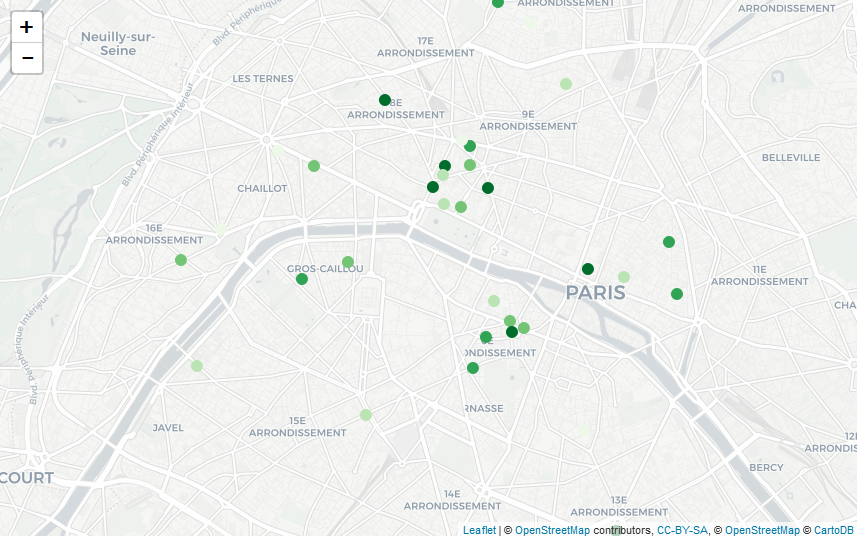Macaron in Paris, France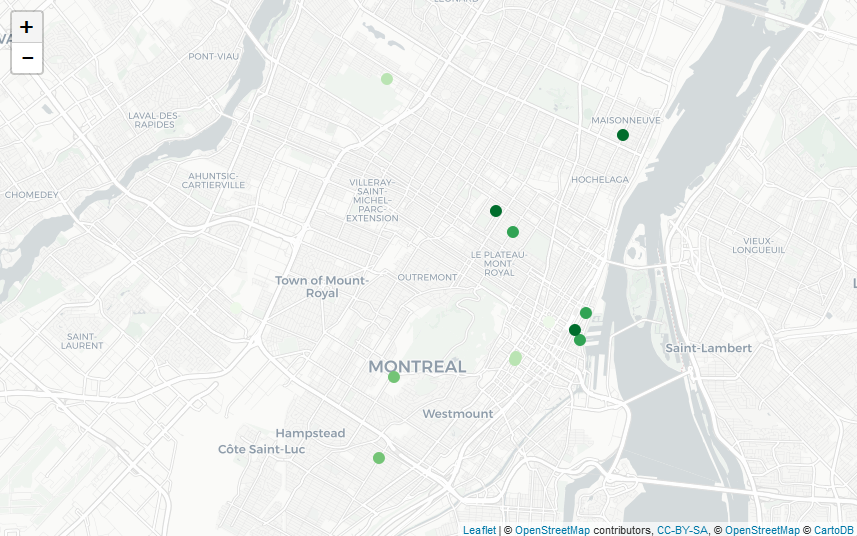Macaron in Montreal, CanadaPoutine in Montreal, CanadaPoutine in Paris, France (I guess French people are not ready for this)

## Weighting tricks for machine learning with Icarus – Part 1

Calibration in survey sampling is a wonderful tool, and today I want to show you how we can use it in some Machine Learning applications, using the R package Icarus. And because ’tis the season, what better than a soccer dataset to illustrate this? The data and code are located on this gitlab repo: https://gitlab.com/haroine/weighting-mlhttps://gitlab.com/haroine/weighting-ml

First, let’s start by installing and loading icarus and nnet, the two packages needed in this tutorial, from CRAN (if necessary):

```install.packages(c("icarus","nnet"))
library(icarus)
library(nnet)
```

Then load the data:

```load("data/weighting_ML_part1.RData")
```

The RData file contains two dataframes, one for the training set and one for the test set. They contain results of some international soccer games, from 01/2008 to 12/2016 for the training set, and from 01/2017 to 11/2017 for the test. Along with the team names and goals scored for each side, a few descriptive variables that we’re going to use as features of our ML models:

```> head(train_soccer)
Date                   team opponent_team home_field elo_team
1 2010-10-12                Belarus       Albania          1      554
2 2010-10-08 Bosnia and Herzegovina       Albania          0      544
3 2011-06-07 Bosnia and Herzegovina       Albania          0      594
4 2011-06-20              Argentina       Albania          1     1267
5 2011-08-10             Montenegro       Albania          0      915
6 2011-09-02                 France       Albania          0      918
opponent_elo importance goals_for goals_against outcome year
1          502          1         2             0     WIN 2010
2          502          1         1             1    DRAW 2010
3          564          1         2             0     WIN 2011
4          564          1         4             0     WIN 2011
5          524          1         2             3    LOSS 2011
6          546          1         2             1     WIN 2011```

elo_team and opponent_elo are quantitative variables indicative of the level of the team at the date of the game ; importance is a measure of high-profile the game played was (a friendly match rates 1 while a World Cup game rates 4). The other variables are imo self-descriptive.

Then we can train a multinomial logistic regression, with outcome being the predicted variable, and compute the predictions from the model:

```outcome_model_unw <- multinom(outcome ~ elo_team + opponent_elo + home_field + importance,
data = train_soccer)

test_soccer\$pred_outcome_unw <- predict(outcome_model_unw, newdata = test_soccer)```

The sheer accuracy of this predictor is kinda good:

```> ## Accuracy
> sum(test_soccer\$pred_outcome_unw == test_soccer\$outcome) / nrow(test_soccer)
 0.5526316```

but it has a problem: it never predicts draws!

```> summary(test_soccer\$pred_outcome_unw)
DRAW LOSS  WIN
0  208  210```

And indeed, draws being less common than other results, it seems more profitable for the algorithm that optimizes accuracy never to predict them. As a consequence, the probabilities of the game being a draw is always lesser than the probability of one team winning it. We could show that the probabilities are not well calibrated.

A common solution to this problem is to use reweighting to correct the imbalances in the sample, which we’ll now tackle. It is important to note that the weighting trick has to happen in the training set to avoid “data leaks”. A very good piece on this subject has been written by Max Kuhn in the documentation of caret.https://topepo.github.io/caret/

Commonly, you would do:

```train_soccer\$weight <- 1
train_soccer[train_soccer\$outcome == "DRAW",]\$weight <- (nrow(train_soccer)/table(train_soccer\$outcome)) * 1/3
train_soccer[train_soccer\$outcome == "LOSS",]\$weight <- (nrow(train_soccer)/table(train_soccer\$outcome)) * 1/3
train_soccer[train_soccer\$outcome == "WIN",]\$weight <- (nrow(train_soccer)/table(train_soccer\$outcome)) * 1/3

```
```> table(train_soccer\$weight)

0.916067146282974  1.22435897435897
3336              1248```

The draws are reweighted with a factor greater than 1 and the other games with a factor lesser than 1. This balances the predicted outcomes and thus improves the quality of the probabilities …

```outcome_model <- multinom(outcome ~ elo_team + opponent_elo + home_field + importance,
data = train_soccer,
weights = train_soccer\$weight)

test_soccer\$pred_outcome <- predict(outcome_model, newdata = test_soccer)
```
```> summary(test_soccer\$pred_outcome)
DRAW LOSS  WIN
96  167  155```

… though at a loss in accuracy:

```> ## Accuracy
> sum(test_soccer\$pred_outcome == test_soccer\$outcome) / nrow(test_soccer)
 0.5263158```

Now let’s look at the balance of our training sample on other variables:

```> round(table(test_soccer\$importance) / nrow(test_soccer),2)

1    2    3    4
0.26 0.08 0.54 0.12
> round(table(train_soccer\$importance) / nrow(train_soccer),2)

1    2    3    4
0.56 0.08 0.23 0.12```

It seems that the test set features a lot more important matches than the training set. Let’s look further, in particular at the dates the matches of the training set were played:

```> round(table(train_soccer\$year) / nrow(train_soccer),2)

2008 2009 2010 2011 2012 2013 2014 2015 2016
0.10 0.11 0.11 0.10 0.11 0.13 0.11 0.11 0.12```

Thus the matches of each year between 2008 and 2016 have the same influence on the final predictor. A better idea would be to give the most recent games a slightly higher influence, for example by increasing their weight and thus reducing the weights of the older games:

```nyears <- length(unique(train_soccer\$year))
year_tweak <- rep(1/nyears,nyears) * 1:nyears
year_tweak <- year_tweak * 1/sum(year_tweak) ## Normalization

```
```> year_tweak
 0.02222222 0.04444444 0.06666667 0.08888889 0.11111111 0.13333333
 0.15555556 0.17777778 0.20000000```

We determine it is thus a good idea to balance on these two additional variables (year and importance). Now how should we do this? A solution could be to create an indicator variable containing all the values of the cross product between the variables outcome, year and importance, and use the same reweighting technique as before. But this would not be very practical and more importantly, some of the sub-categories would be nearly empty, making the procedure not very robust. A better solution is to use survey sampling calibration and Icarus 🙂

```train_soccer\$weight_cal <- 1
importance_pct_test <- unname(
table(test_soccer\$importance) / nrow(test_soccer),
)

marginMatrix <- matrix(, nrow = 0, ncol = 1) %>% ## Will be replaced by newMarginMatrix() in icarus 0.3.2
addMargin("outcome", c(0.333,0.333,0.333)) %>%
addMargin("importance", importance_pct_test) %>%
addMargin("year", year_tweak)

train_soccer\$weight_cal <- calibration(data=train_soccer, marginMatrix=marginMatrix,
colWeights="weight_cal", pct=TRUE, description=TRUE,
popTotal = nrow(train_soccer), method="raking")

outcome_model_cal <- multinom(outcome ~ elo_team + opponent_elo + home_field + importance,
data = train_soccer,
weights = train_soccer\$weight_cal)

test_soccer\$pred_outcome_cal <- predict(outcome_model_cal, newdata = test_soccer)```

icarus gives a summary of the calibration procedure in the log (too long to reproduce here). We then observe a slight improvement in accuracy compared to the previous reweighting technique:

```> sum(test_soccer\$pred_outcome_cal == test_soccer\$outcome) / nrow(test_soccer)
 0.5478469```

But more importantly we have reason to believe that the we improved the quality of the probabilities assigned to each event (we could check this using metrics such as the Brier score or calibration plots) 🙂

It is also worth noting that some algorithms (especially those who rely on bagging, boosting, or more generally on ensemble methods) naturally do a good job at balancing samples. You could for example rerun the whole code and replace the logit regressions by boosted algorithms. You would then observe fewer differences between the unweighted algorithm and its weighted counterparts.

Stay tuned for the part 2, where we’ll show a trick to craft better probabilities (particularly for simulations) using external knowledge on probabilities.